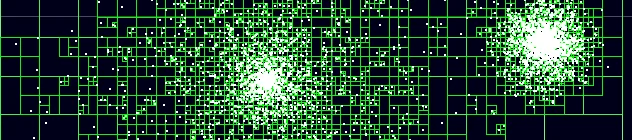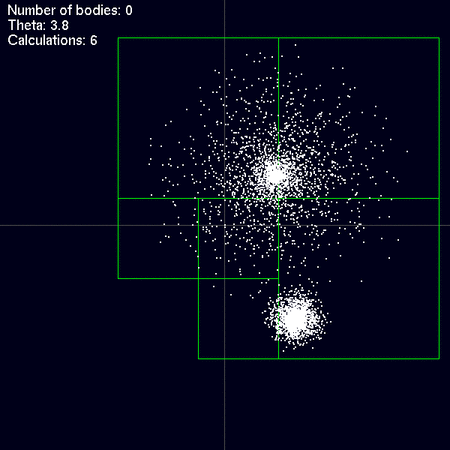# The Barnes-Hut Galaxy Simulator## The N-Body Problem

This article explains how to use the Barnes-Hut algorithm for effectively solving N-Body problems. So what is an N-Body Problem? Let's assume a region of space contains an arbitrary number of N bodies. Each body has its own potential and a resulting force field. This could be charges causing an electrical field (Coulomb's law) or planets in space (Law of Gravity). The potential of the bodies can be summed up resulting in a combined potential field which depends on the location of each of the bodies and its physical properties (i.e. mass or charge). According the Newtons first law the bodies themself will experience an acceleration caused by the field. As a result there is a force field which depends on the locations of the n-nodies whilst the location of the body depends on the force field. Mathematically this problem can be described with a system of differential equations of second order.

When solving such problems one usually starts with a known initial distribution of the bodies and known velocities and accelerations. From the initial conditions the system can be advanced in time by using numerical computations and a suitable integration scheme. Typical problems of this kind are for instance the computation of galaxy evolution in astrophysics or the behaviour of molecules in biology.

Setting up the equationts of motion is pretty simple. The equation for computing the force caused by number of particles on a single particle can be computed by the law of gravity:

\begin{equation} m_i \ddot{\vec{r_i}} = G \sum_{j=1;j \ne i}^N \Big( m_i m_j \frac{ \vec{r_i} - \vec{r_j}} {|\vec{r_i} - \vec{r_j}|^3} \Big) \end{equation}

with:

• $$m_i, m_j$$ - Mass of the particles i and j
• $$\vec{r_i}, \vec{r_j}$$ - Positional vectors of the particles
• $$G$$ - Gravitational constant

Lets assume Fij is the force acting between particles i and j. The total number of force calculations required to compute the state of the system is N*(N-1). According to Newtons third law every force has an opposite force equal to itself: Fij = -Fji. The total number of force calculations is therefore:

\begin{equation} {1 \over 2 } N (N-1) \approx O(N^2) \end{equation}

The problem is of order O(N2). If the number of particles doubles, the number of calculations quadrupels. If the number of particles is increased by factor ten, the number of calculations increases by a factor of 100 and so on... From this simple relation it is clear that computing the N-Body problem for large numbers of particles will quickly become very costly in terms of computational power. A more effective algorithm, which scales better with an increasing number of particles is needed.

## The Barnes-Hut Algorithm

The Barnes-Hut Algorithm describes an effective method for solving n-body problems. It was originally published in 1986 by Josh Barnes and Piet Hut . Instead of directly summing up all forces, it is using a tree based approximation scheme which reduces the computational complexity of the problem from O(N2) to O(N log N).

It works by reducing the number of force calculations by grouping particles. The basic idea behind the algorithm is that the force which a particle group excerts on a single particle can be approximated by the force of a pseudo particle located at the groups center of mass. For instance, the force which the Andromeda galaxy excerts on the milky way can be approximated by a point mass located at the centre of the Andromeda galaxy. There is no need to integrate over all stars in the Andromeda galaxy provided the distance between the two galaxies is large enough. This approximation is valid as long as the distance from a point group to a particle is large and the radius of the group is small in relation to the distance between the group and the particle. The ratio of group distance (d) and group radius (r) is called the Multipole-Acceptance-Criterion (MAC):

$$\theta = {d \over r }$$

If theta drops below a certain threshold the quality of the approximation starts to deteriorate, resulting in larger errors. Barnes and Hut use a value of θ=1. The general rule is: The smaller θ, the better the simulation results. The Barnes-Hut algorithm applies this basic principle by subdividing the space of the simulation domain recursively (see Image 2).Image 1: The force a particle group excerts on a single particle at distance r can be approximated by the force of a point mass, provided the distance between the group and the particle is large in comparison with the group size d.Image 2: The gravitational effect excerted by the star cluster and the single star B on star A can be approximated by a point mass. On a smaller scale the gravitational effect of the cluster on star B can also be approximated by a point mass since the distance of B from the cluster is much larger compared to the size of the cluster (r2 >> d2).

## Implementation

The following section will explain the algorithm. All explanations and examples are simplified and deal with a two dimensional domain. Extending the concept to a third dimension is pretty straightforward but not the scope of this article.

### The tree structureImage 3: right: Spacial distributiong of particles in their quadrants; left: The tree structure of the distribution.

The creation of the quadtree is a recursive process. It can be described by the following pseudocode:

Function MainApp::BuildTree
{
ResetTree

for all particles
rootNode->InsertToNode(particle)
end for
}

Function TreeNode::InsertToNode(newParticle)
{
if number of particles in this node > 1
{

}
else if number of particles in this node == 1
{

}
else if node is empty
{
store newParticle in node as existingParticle
}

Increase number of particles
}

The tree has to be recreated for each step of the simulation. The computational cost of recreating the quadtree depends on the distribution of the particles (see ). The cost of adding a particle to the tree is proportional to its distance from the root node. Distributions with many densly packed particles require more operations because the tree must be subdivided often to place all particles in their own subdivided quadrant.

### Computing the mass distribution

Up until now the tree only contains the spacial distribution of the particles. The next step deals with calculating the mass distribution of the quadtree (or octtree). It consists of computing the total mass of all particles contained in the child nodes of each tree node as well as their center of mass. This data must be computed for each tree node. The calculation can be implemented recursively by scanning all child nodes of each treenode. The following pseudo code illustrates this procedure:

Function TreeNode::ComputeMassDistribution()
{
if number of particles in this node equals 1
{
CenterOfMass = Particle.Position
Mass = Particle.Mass
}
else
{
// Compute the center of mass based on the masses of
// all child quadrants and the center of mass as the
// center of mass of the child quadrants weightes with
// their mass
for all child quadrants that have particles in them
{
}
CenterOfMass /= Mass
}
}

The procedure is of order O(N log N) .

### Force calculation

The last step of the algorithm is computing the forces excerted on the particles. This is what makes the Barnes-Hut algorithm faster than the direct summation of all mutual forces. Do you remember the images 1 and 2 ? This is the basic principle we are going to implement here. In order to computer the force excerted on a single particle the quadtree must again be iterated recursively. For each node θ is computed:

$$\theta = {d \over r }$$ where:
• d - Size of the box
• r - Particle distance from the nodes center of mass

If θ lies below a certain threshold the force can be approximated by inserting the quadrant nodes mass and its center of mass in the law of gravity. The child nodes don't have to be summed up separately. A reasonable criterion for this decision is θ < 1. If θ is larger than the threshold the quadrants effect can't be approximated and all of its child nodes have to be tested again. The iteration stops only after all nodes have been tested. The worst case scenario is having to test all nodes without finding any node that meets the MAC. In such a case the result is similar to summing up all mutual particle forces (θ=0). The iteration depth can be finetuned by adjusting θ. Animation 1 illustrates the influence of θ on the number of force computations.Animation 1: The animation shows a distribution of 5000 particles. The quadrants shown are the ones that are used for calculating the force excerted on the particle at the origin of the coordinate system. The higher θ is, the fewer the number of nodes that are necessary for the force calculation (and the larger the error).

Finally the pseude code for the force calculation:


Function MainApp::CalcForce
for all particles
force = RootNode.CalculateForceFromTree(particle)
end for
end

Function force = TreeNode::CalculateForce(targetParticle)
force = 0

if number of particle equals 1
force = Gravitational force between targetParticle and particle
else
r = distance from nodes center of mass to targetParticle
d = height of the node
if (d/r < θ)
force = Gravitational force between targetParticle and node
else
for all child nodes n
force += n.CalculateForce(particle)
end for
end if
end
end

## Colliding galaxies

By combining the Barnes-Hut-Algorithm with a suitable integration scheme (i.e. Adams-Bashforth) one can relatively easy compute a two dimensional galaxy collision:

In order to keep the galaxies together it is assumed that both of them contain a supermassive black hole in their center and lots of stars orbitting it.